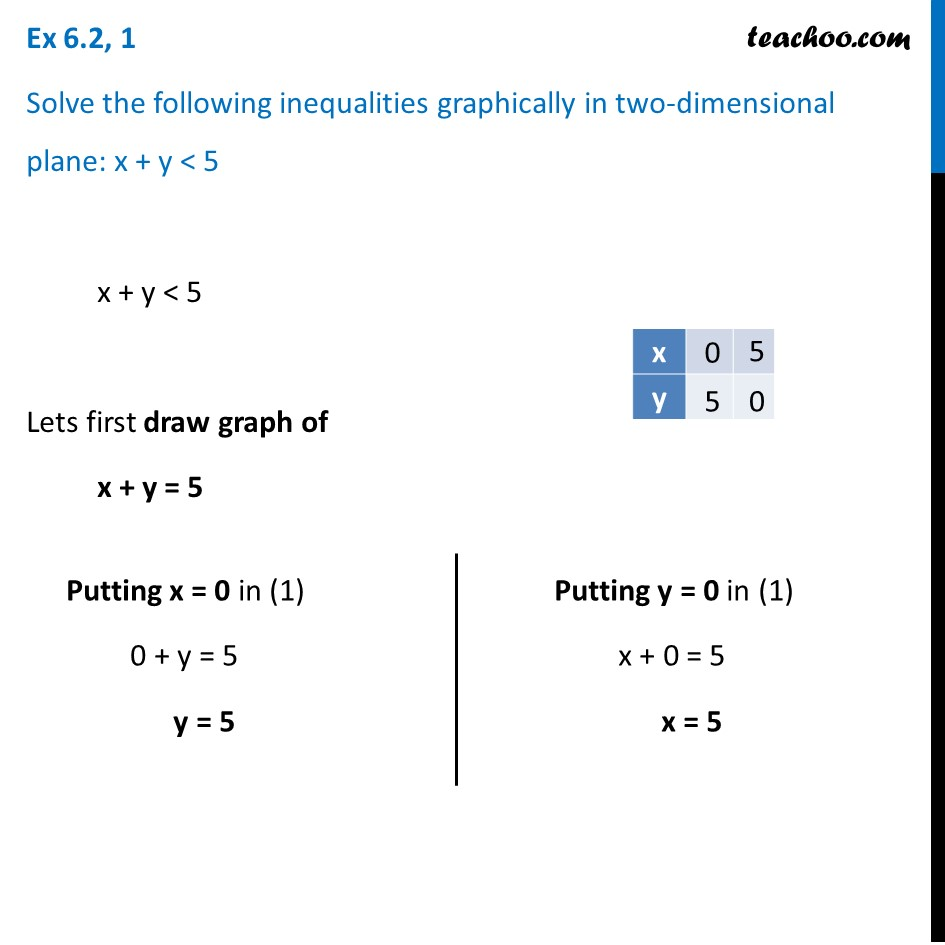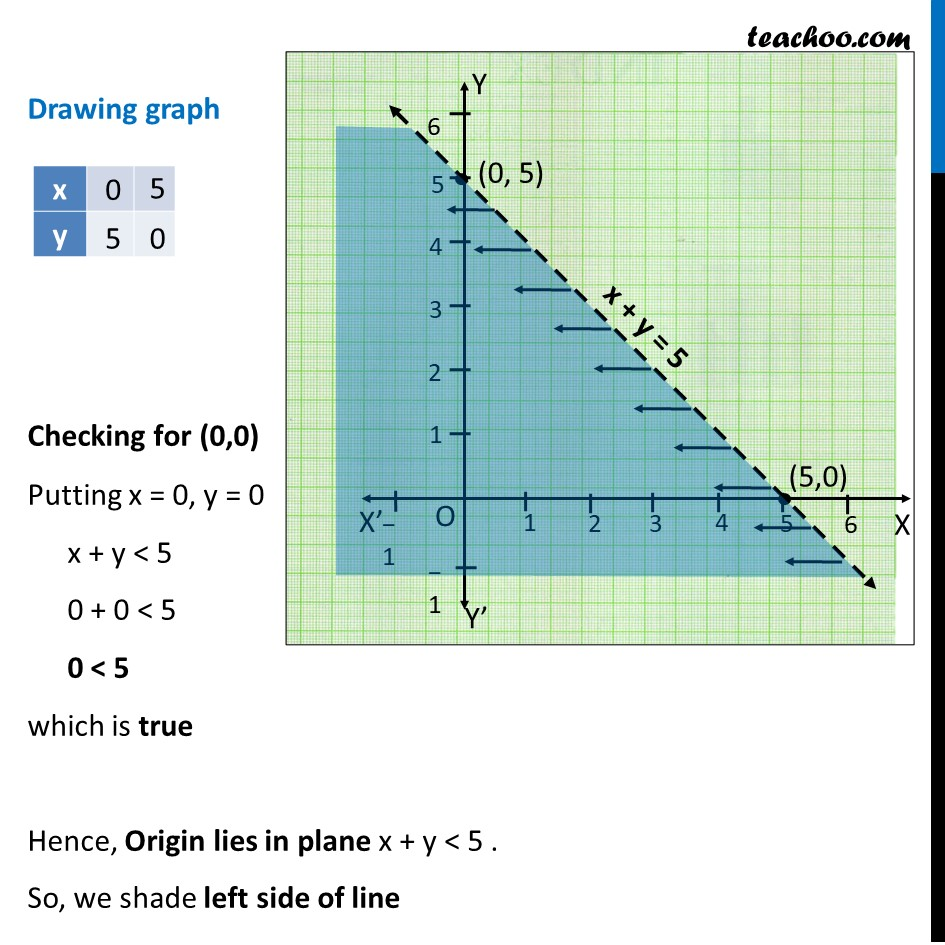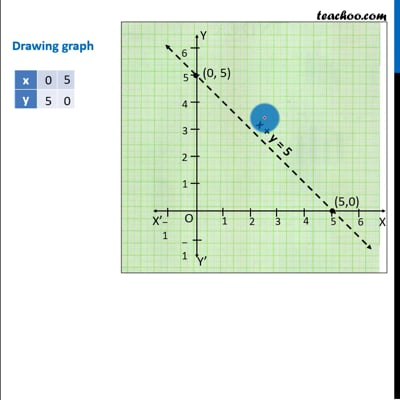Ex 6.2

Chapter 6 Class 11 Linear Inequalities (Term 2)
Serial order wiseThis video is only available for Teachoo black users

### Transcript

Ex 6.2, 1 Solve the following inequalities graphically in two-dimensional plane: x + y < 5 x + y < 5 Lets first draw graph of x + y = 5 Putting x = 0 in (1) 0 + y = 5 y = 5 Putting y = 0 in (1) x + 0 = 5 x = 5 Drawing graph Checking for (0,0) Putting x = 0, y = 0 x + y < 5 0 + 0 < 5 0 < 5 which is true Hence, Origin lies in plane x + y < 5 . So, we shade left side of line